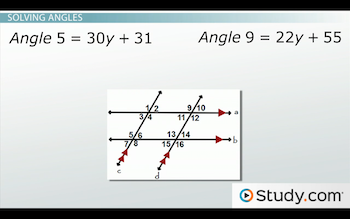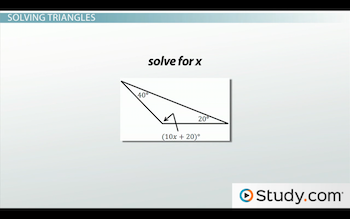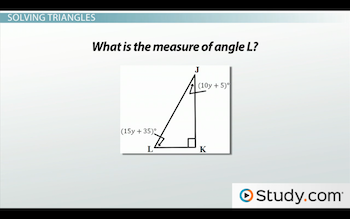# Angles and Triangles: Practice Problems

An error occurred trying to load this video.

Try refreshing the page, or contact customer support.

Coming up next: Properties of Shapes: Circles

### You're on a roll. Keep up the good work!

Replay
Your next lesson will play in 10 seconds
• 0:08 Solving Angles
• 3:29 Solving Triangles
• 6:54 Lesson Summary

Want to watch this again later?

Timeline
Autoplay
Autoplay
Speed

#### Recommended Lessons and Courses for You

Lesson Transcript
Instructor: DaQuita Hester

DaQuita has taught high school mathematics for six years and has a master's degree in secondary mathematics education.

Want more practice solving with angle pairs? How about more review for solving angles in triangles? Look no further. Get more practice here, and test your ability with a quiz.

## Solving Angles

In another lesson, we learned about the different types of angles: consecutive interior, alternate interior, alternate exterior and corresponding. We discovered that when two lines are parallel, all of the angle pairs are congruent, except for consecutive interior angles, which are supplementary. We also learned about vertical angles, which are always congruent. Let's do some practice with these angles.

In the figure below, let Angle 5 = 30y + 31, and let Angle 9 = 22y + 55. What is the value of y?Angle 5 and Angle 9 don't match any of the angle pairs, so let's find the connection between their measures. We notice that Angle 5 corresponds to Angle 1, and Angle 1 corresponds to Angle 9. Knowing that all corresponding angles are congruent, Angle 5 = Angle 1, and Angle 1 = Angle 9. So, by the transitive property of equality, we can conclude that Angle 5 = Angle 9. By substituting the equations, we have 30y + 31 = 22y + 55. From here, we can subtract 31 from both sides to get 30y = 22y + 24, and then subtract 22y from each side to get 8y = 24. To finish, we will divide both sides by 8 to determine that y = 3.

Let's do another using the same figure. This time, let Angle 4 = 14x - 23, and let Angle 14 = 4x + 5. Find the measure of Angle 15.

Once again, these angles are not a special angle pair; so, let's find the connection. Angle 4 corresponds to Angle 12, and Angle 12 is consecutive interior to Angle 14. Therefore, Angle 4 = Angle 12, and Angle 12 + Angle 14 = 180. With this knowledge, we can replace Angle 12 with Angle 4 to get Angle 4 + Angle 14 = 180. With the equations, we have 14x - 23 + 4x + 5 = 180. Combining like terms gives us 18x - 18 = 180, and then, by adding 18 to both sides, we get 18x = 198. Last, we will divide both sides by 18 to conclude that x = 11.

Now we can find the value of Angle 15, which is vertical to and congruent with Angle 14. Substituting 11 into the equation, we see that Angle 14 = 4(11) + 5, which equals 49. Therefore, we can also conclude that Angle 15 = 49 degrees.

## Solving Triangles

When working with triangles, remember that the sum of all three angles in every triangle is 180 degrees. Let's get started.

In this first triangle below, let's solve for x.For each angle, we either have a measure or an equation. For that reason, let's add all of the angles together to equal 180 degrees. Doing so, we have 40 + 10x + 20 + 20 = 180, and by combining like terms, we have 10x + 80 = 180. Next, let's subtract 80 from both sides to get 10x = 100, and then let's divide each side by 10 to finish with x = 10.

Here's another. This is triangle JKL. What is the measure of Angle L?To unlock this lesson you must be a Study.com Member.

### Register to view this lesson

Are you a student or a teacher?

### Unlock Your Education

#### See for yourself why 30 million people use Study.com

##### Become a Study.com member and start learning now.
Back
What teachers are saying about Study.com

### Earning College Credit

Did you know… We have over 160 college courses that prepare you to earn credit by exam that is accepted by over 1,500 colleges and universities. You can test out of the first two years of college and save thousands off your degree. Anyone can earn credit-by-exam regardless of age or education level.